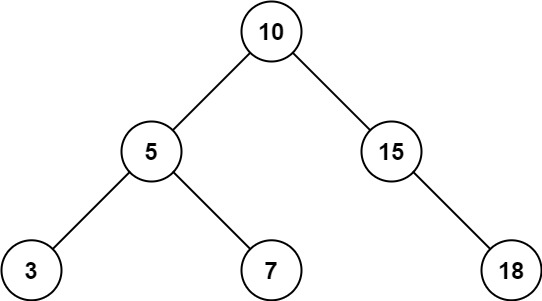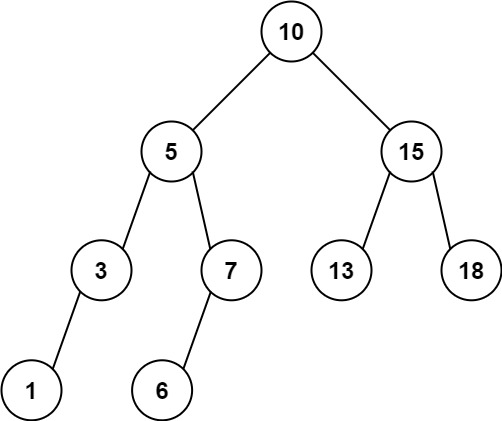# 938. Range Sum of BST

catepk·2021년 8월 22일
0

## LeetCode

목록 보기
52/88938. Range Sum of BST

### 문제

Given the root node of a binary search tree and two integers low and high, return the sum of values of all nodes with a value in the inclusive range [low, high].

Example 1:Input: root = [10,5,15,3,7,null,18], low = 7, high = 15
Output: 32
Explanation: Nodes 7, 10, and 15 are in the range [7, 15]. 7 + 10 + 15 = 32.

Example 2:Input: root = [10,5,15,3,7,13,18,1,null,6], low = 6, high = 10
Output: 23
Explanation: Nodes 6, 7, and 10 are in the range [6, 10]. 6 + 7 + 10 = 23.

Constraints:

The number of nodes in the tree is in the range [1, 2 * 10^4].
1 <= Node.val <= 10^5
1 <= low <= high <= 10^5
All Node.val are unique.

### 풀이

-----Brute Force-----

# Definition for a binary tree node.
# class TreeNode:
#     def __init__(self, val=0, left=None, right=None):
#         self.val = val
#         self.left = left
#         self.right = right
class Solution:
def rangeSumBST(self, root: Optional[TreeNode], low: int, high: int) -> int:
if not root:
return 0
return (root.val if low <= root.val <= high else 0) + self.rangeSumBST(root.left, low, high) + self.rangeSumBST(root.right, low, high)

-----DFS-----

# Definition for a binary tree node.
# class TreeNode:
#     def __init__(self, val=0, left=None, right=None):
#         self.val = val
#         self.left = left
#         self.right = right
class Solution:
def rangeSumBST(self, root: Optional[TreeNode], low: int, high: int) -> int:
def dfs(node: TreeNode):
if not node:
return 0
if node.val < low:
return dfs(node.right)
elif node.val > high:
return dfs(node.left)
return node.val + dfs(node.left) + dfs(node.right)
return dfs(root)

-----DFS-----

# Definition for a binary tree node.
# class TreeNode:
#     def __init__(self, val=0, left=None, right=None):
#         self.val = val
#         self.left = left
#         self.right = right
class Solution:
def rangeSumBST(self, root: Optional[TreeNode], low: int, high: int) -> int:
stack = [root]
sums = 0
while stack:
node = stack.pop()
if node:
if node.val > low:
stack.append(node.left)
if node.val < high:
stack.append(node.right)
if low <= node.val <= high:
sums += node.val
return sums

-----BFS-----

# Definition for a binary tree node.
# class TreeNode:
#     def __init__(self, val=0, left=None, right=None):
#         self.val = val
#         self.left = left
#         self.right = right
class Solution:
def rangeSumBST(self, root: Optional[TreeNode], low: int, high: int) -> int:
stack = [root]
sums = 0
while stack:
node = stack.pop(0)
if node:
if node.val > low:
stack.append(node.left)
if node.val < high:
stack.append(node.right)
if low <= node.val <= high:
sums += node.val
return sums